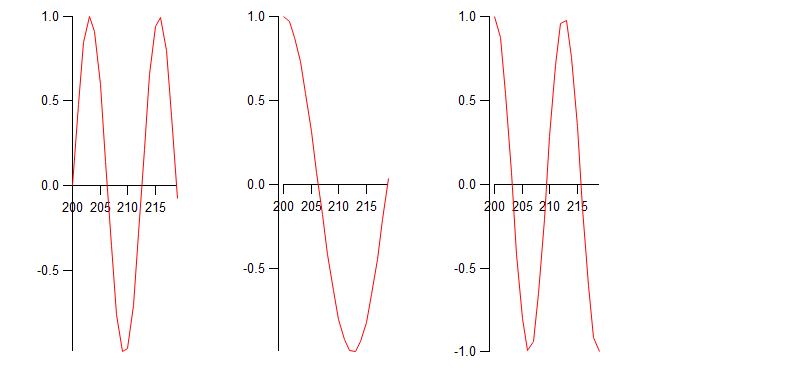Does 'ModifyGraph standoff=0' work for multiple axes?I am attempting to use the command "ModifyGraph standoff=0" to remove the gap between multiple left and bottom axes in a plot of mine, but it doesn't work as expected. Here's a sample image I generated from the code below:I have 3 sets of axes in one graph window - does the standoff command not work in this case? The documentation For ModifyGraph says "If '(axisName)' is omitted, all axes in the graph are affected. For instance, 'ModifyGraph standoff=0' disables axis standoff for all axes in the graph."

Furthermore, when I try to use the ModifyAxis GUI by clicking on the axes and manually checking / unchecking the Standoff checkbox, it has no effect.

The code below should generate the same issue I'm seeing. Is this expected behaviour? Thanks in advance for any insight.

Function StandoffDoesntWork()
// create test waves
NewDataFolder/O/S root:tests
Make/O/N=20 a = sin(x/2)
Make/O/N=20 b = cos(x/4)
Make/O/N=20 c = cos(x/2)
Make/O/N=20 timew

// populate the fake timewave; its range is from 200-219
int i = 0
for(i=0; i < numpnts(timew); i++)
timew[i] = i + 200
endfor

// create 3 sets of axes to plot the 3 graphs
// the positions of the bottom and left axes intersect each other at zero (0) with the freePos command
Display/W=(294,375,897,651)
AppendToGraph/L=L1/B=B1 a vs timew
ModifyGraph axisEnab(B1)={0,0.15},freePos(L1)={0,B1},freePos(B1)={0,L1}
AppendToGraph/L=L2/B=B2 b vs timew
ModifyGraph axisEnab(B2)={0.3,0.45},freePos(L2)={0,B2},freePos(B2)={0,L2}
AppendToGraph/L=L3/B=B3 c vs timew
ModifyGraph axisEnab(B3)={0.6,0.75},freePos(L3)={0,B3},freePos(B3)={0,L3}

// attempt to get rid of the gap by setting standoff=0
ModifyGraph standoff=0

SetDataFolder root:
End

It's confusing- Standoff doesn't apply to free axes, instead you use one of the positioning settings. Since it became available, I vastly prefer setting "Free Position" to "Fraction of PlotArea". I set your Ln axes to use that positioning mode, with appropriate offsets depending on the Bn axis Draw Between settings. Here is the recreation macro from my modifications:

Window Graph0() : Graph
PauseUpdate; Silent 1       // building window...
String fldrSav0= GetDataFolder(1)
SetDataFolder root:tests:
Display /W=(294,375,897,651)/L=L1/B=B1 a vs timew
AppendToGraph/L=L2/B=B2 b vs timew
AppendToGraph/L=L3/B=B3 c vs timew
SetDataFolder fldrSav0
ModifyGraph standoff=0
ModifyGraph freePos(L1)={0,kwFraction}
ModifyGraph freePos(B1)={0,L1}
ModifyGraph freePos(L2)={0.3,kwFraction}
ModifyGraph freePos(B2)={0,L2}
ModifyGraph freePos(L3)={0.6,kwFraction}
ModifyGraph freePos(B3)={0,L3}
ModifyGraph axisEnab(B1)={0,0.15}
ModifyGraph axisEnab(B2)={0.3,0.45}
ModifyGraph axisEnab(B3)={0.6,0.75}
EndMacro

Thanks John! I didn't know that was the case for free axes - I applied your solution to my case and it worked well.

What makes an axis considered "free"? Is it just any axis that has been modified by the freePos command, or is it any additional axis that is not the default "left"/"bottom" etc.?

Free axes are ones that are not left, right, bottom or top. They are made with, for instance, Display/L=<free axis name>. They are "free" in the sense that they can be positioned freely anywhere within the graph area.